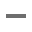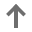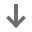# Change Signature

The Change Signature refactoring combines several different modifications that can be applied to a function signature. You can use this refactoring for the following purposes:

• To change the function name.

• To add new parameters and remove the existing ones.

• To assign default values to the parameters.

• To reorder parameters.

When changing a function signature, PyCharm searches for all usages of the function and updates all the calls, implementations, and override replacements of the function that can be safely modified to reflect the change.

## Examples

Before

After

```# This function will be renamed: def fibonacci( n ): a, b = 0, 1 while b < n: print( b ) a, b = b, a+b n = int(input("n = ")) fibonacci( n )```

```# Function with the new name: def fibonacci_numbers( n ): a, b = 0, 1 while b < n: print( b ) a, b = b, a+b n = int(input("n = ")) fibonacci_numbers( n )```

```# New parameters will be added: def fibonacci( n ): a, b = 0, 1 while b < n: print( b ) a, b = b, a+b n = int(input("n = ") fibonacci( n )```

```# Function with the new parameters. # Do not forget to specify the default values of the parameters, which will be used in the function call. def fibonacci( n,a,b ): a, b = 0, 1 # this should be done manually! while b < n: print( b ) a, b = b, a+b n = int(input("n = ") fibonacci( n,0,1 )```

## Default value and propagation of new parameters

For each new parameter added to a function, you can specify:

• A default value (or an expression) (the Default value field).

You can also propagate the parameters you have introduced to the functions that call the function whose signature you are changing.

The refactoring result depends on whether you specify the default value and whether you use propagation.

Propagation. New parameters can be propagated to any function that calls the function whose signature you are changing. In such case, generally, the signatures of the calling functions change accordingly.
These changes, however, also depend on the default values set for the new parameters.

Default value. Generally, this is the value to be added to the function calls.

If the new parameter is not propagated to a calling function, the calls within such function will also use this value.

If propagation is used, this value won't affect the function calls within the calling functions.

## Changing a function signature

1. In the editor, place the cursor within the name of the function whose signature you want to change.

2. Do one of the following:
• Press Ctrl+F6.

• Choose Refactor | Change Signature on the main menu or

• Choose Refactor | Change Signature on the context menu.

3. In the Change Signature dialog, make the necessary changes to the function signature and specify what other, related, changes are required.

You can:

• Change the function name. To do that, edit the text in the Name field.

• Manage the function parameters using the table and the buttons in the Parameters area:
• To add a new parameter, clickand specify the properties of the new parameter in the corresponding table row.

• To remove a parameter, click any of the cells in the corresponding row, and then click.

• To reorder the parameters, use theandbuttons. For example, if you want to put a certain parameter first in the list, click any of the cells in the row corresponding to that parameter, and then clickthe required number of times.

• To change the name or the default value of a parameter, make the necessary updates in the table of parameters (in the fields Name and Default value respectively).

4. To perform the refactoring right away, click Refactor.

To see the expected changes and make the necessary adjustments prior to actually performing the refactoring, click Preview.